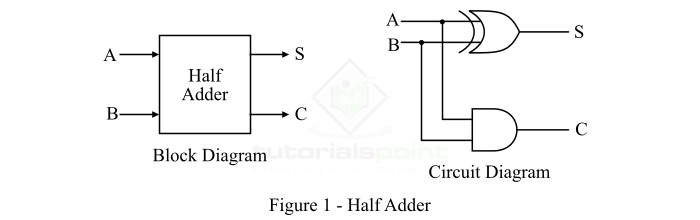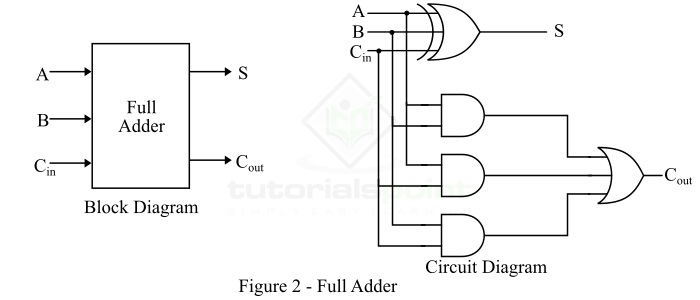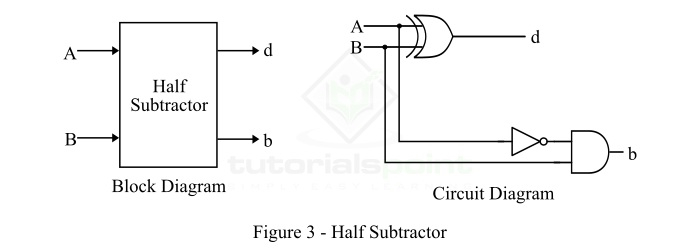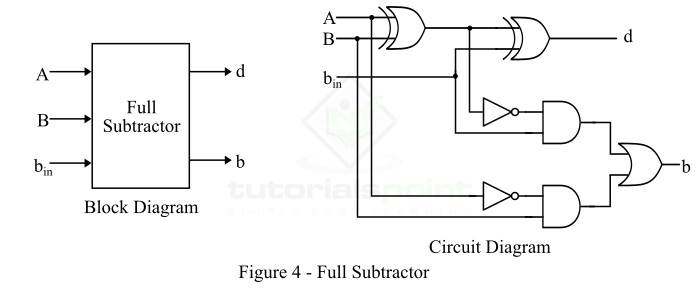# Adders and Subtractors in Digital Electronics

In digital electronics, adders and subtractors both are the combinational logic circuits (a combinational logic circuit is one whose output depends only on the present inputs, but not on the past outputs) that can add or subtract numbers, more specifically binary numbers. Adders and subtractors are the crucial parts of arithmetic logic circuits in processing devices like microprocessors or microcontrollers. In this article, we will discuss adders and subtractors in detail.

We have different types of digital devices like computers, calculators that can perform a variety of processing functions like addition, subtraction, multiplication, division, etc. The most basic arithmetic operation that the ALU (arithmetic logic unit) of a computer performs is the addition of two or more binary numbers. To perform the operation of addition, a combinational logic circuit, named Adder is used.

Adders are classified into two types namely −

A half-adder is a combinational logic circuit that performs the addition of only two bits (binary digits). Whereas, a full-adder is a combination circuit that performs three bits (binary digits), where two are the significant bits and one is a carry from previous execution.

A combinational logic circuit which is designed to add two binary digits is called as a half adder. The half adder provides the output along with a carry value (if any). The half adder circuit is designed by connecting an EX-OR gate and one AND gate. It has two input terminals and two output terminals for sum and carry. The block diagram and circuit diagram of a half adder are shown in Figure-1.In the case of a half adder, the output of the EX-OR gate is the sum of two bits and the output of the AND gate is the carry. Although, the carry obtained in one addition will not be forwarded in the next addition because of this it is known as half adder.

### Truth Table of Half Adder

The following is the truth table of the half-adder −

Inputs Outputs
A B S (Sum) C (Carry)
0 0 0 0
0 1 1 0
1 0 1 0
1 1 0 1

The characteristic equations of half adder, i.e., equations of sum (S) and carry (C) are obtained according to the rules of binary addition. These equations are given below −

The sum (S) of the half-adder is the XOR of A and B. Thus,

$$\mathrm{Sum,\, S=A\oplus B=AB'+A'B }$$

The carry (C) of the half-adder is the AND of A and B. Therefore,

$$\mathrm{Carry,\, C=A\cdot B }$$

## What is a Full Adder?

A combinational logic circuit that can add two binary digits (bits) and a carry bit, and produces a sum bit and a carry bit as output is known as a full-adder.

In other words, a combinational circuit which is designed to add three binary digits and produces two outputs (sum and carry) is known as a full adder. Thus, a full adder circuit adds three binary digits, where two are the inputs and one is the carry forwarded from the previous addition. The block diagram and circuit diagram of the full adder are shown in Figure-2.Hence, the circuit of the full adder consists of one EX-OR gate, three AND gates and one OR gate, which are connected together as shown in the full adder circuit in Figure-2.

### Truth Table of Full Adder

The following is the truth table of the full-adder circuit −

Inputs Outputs
A B Cin S (Sum) C (Carry)
0 0 0 0 0
0 0 1 1 0
0 1 0 1 0
0 1 1 0 1
1 0 0 1 0
1 0 1 0 1
1 1 0 0 1
1 1 1 1 1

Hence, from the truth table, it is clear that the sum output of the full adder is equal to 1 when only 1 input is equal to 1 or when all the inputs are equal to 1. While the carry output has a carry of 1 if two or three inputs are equal to 1.

### Characteristic Equations of Full Adder

The characteristic equations of the full adder, i.e. equations of sum (S) and carry output (Cout) are obtained according to the rules of binary addition. These equations are given below −

The sum (S) of the full-adder is the XOR of A, B, and Cin. Therefore,

$$\mathrm{Sum,\, S=A\oplus B\oplus C_{in}=A'B'C_{in}+A'BC'_{in}+AB'C'_{in}+ABC_{in} }$$

The carry (C) of the half-adder is the AND of A and B. Therefore,

$$\mathrm{Carry,\, C_{out}=AB+AC_{in}+BC_{in}}$$

## What is a Subtractor?

The subtraction of two binary numbers can be performed by taking the 1’s or 2’s complement of the inputs. By this method, the subtraction operation becomes an addition operation, and thus can be performed by using the adder circuits. This results in the reduction of hardware and cost.

In the subtraction operation, each subtrahend bit (B) of the number is subtracted from its corresponding significant minuend bit (A) to obtain a difference bit.

Similar to adders, subtractors are also of two types −

• Half Subtractor
• Full Subtractor

Let us discuss the half-subtractor and full-subtractor in detail.

## What is a Half-Subtractor?

A half-subtractor is a combinational logic circuit that have two inputs and two outputs (i.e. difference and borrow). The half subtractor produces the difference between the two binary bits at the input and also produces a borrow output (if any). In the subtraction (A-B), A is called as Minuend bit and B is called as Subtrahend bit. The block diagram and logic circuit diagram of the half subtractor is shown in Figure-3.Hence, from the logic circuit diagram, it is clear that a half subtractor can be realized using an XOR gate together with a NOT gate and an AND gate.

### Truth Table of Half Subtractor

The following is the truth table the half-subtractor −

Inputs Outputs
A B d (Difference) b (Borrow)
0 0 0 0
0 1 1 1
1 0 1 0
1 1 0 0

### Characteristic Equation of Half Subtractor

The characteristic equations of the half subtractor, i.e. equations of the difference (d) and borrow output (b) are obtained by following the rules of binary subtraction. These equations are given below −

The difference (d) of the half subtractor is the XOR of A and B. Therefore,

$$\mathrm{Difference,\, d=A\oplus B=A'B+AB'}$$

The borrow (b) of the half subtractor is the AND of A’ (compliment of A) and B. Therefore,

$$\mathrm{Borrow,\, b=A'B}$$

## What is a Full-Subtractor?

A full-subtractor is a combinational circuit that has three inputs A, B, bin and two outputs d and b. Where, A is the minuend, B is subtrahend, bin is borrow produced by the previous stage, d is the difference output and b is the borrow output.

Since, the half subtractor can only be used to find the difference of LSBs (Least Significant Bits) of two binary numbers. Thus, if there is any borrow during the subtraction of the LSBs, it will affect the subtraction of the next bits of numbers. To overcome this problem of the half subtractor, a full subtractor is realized. The block diagram and circuit diagram of a fullsubtractor is shown in Figure-4.Therefore, we can realize the full-subtractor using two XOR gates, two NOT gates, two AND gates, and one OR gate.

### Truth Table of Full-Subtractor

The following is the truth table of the full-subtractor −

Inputs Outputs
A B bin d (Difference) b (Borrow)
0 0 0 0 0
0 0 1 1 1
0 1 0 1 1
0 1 1 0 1
1 0 0 1 0
1 0 1 0 0
1 1 0 0 0
1 1 1 1 1

### Characteristic Equations of Full Subtractor

The characteristic equations of the full subtractor, i.e. equations of the difference (d) and borrow output (b) are obtained by following the rules of binary subtraction. These equations are given below −

The difference (d) of the full subtractor is the XOR of A, B, and bin. Therefore,

$$\mathrm{Difference,\, d=A\oplus B\oplus b_{in}=A'B'b_{in}+AB'b'_{in}+A'Bb'_{in}+ABb_{in}}$$

The borrow (b) of the full subtractor is given by,

$$\mathrm{Borrow,\, b=A'B'b_{in}+A'Bb'_{in}+A'Bb_{in}+ABb_{in}}$$

Or

$$\mathrm{Borrow,\, b=A'B\left ( b_{in}+b'_{in} \right )+\left (AB+A'B' \right )b_{in}=A'B+\left (A\oplus B \right )'b_{in}}$$

## Conclusion

In this tutorial, we discussed in detail about adders and subtractors in digital electronics. We may conclude the above discussion in the following points −

• A combinational logic circuit which is designed to add two binary digits is called as a half adder.

• A combinational logic circuit that can add two binary digits (bits) and a carry bit, and produces a sum bit and a carry bit as output is known as a full-adder.

• A half-subtractor is a combinational logic circuit that have two inputs and two outputs (i.e. difference and borrow).

• A full-subtractor is a combinational circuit that has three inputs A, B, bin and two outputs d and b.

Both adders and subtractors are the crucial logic circuits in arithmetic logic units of microprocessors and microcontrollers. This is because, they perform the two most basic arithmetic operations, i.e., addition and subtraction of binary numbers. Another important point to note about the adder and subtractor is that they both are the combinational logic circuits, i.e., their outputs depend on the present inputs only, but not on the past history.